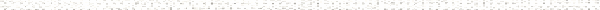A Precalculus OutlineObjective: Given information about a function write an expression for f(x) and find its domain.Objective: Given a function, evaluate the function at a specified value and simplify the result.Objective: Given a piecewise-defined function, evaluate the function at a specified value and simplify the result.Objective: Given a function, write its implied domain using interval notation.Objective: Apply the substitution principle to evaluate expressions.Objective: Simplify numerical and algebraic expressions.Objective: Given a rational expression, correctly determine which numbers must be excluded to prevent division by zero.Objective: Given a radical expression, determine which numbers must be included to produce real number results.Objective: Given functions f(x) and g(x), write (f+g)(x) and state its domain using interval notation.Objective: Given functions f(x) and g(x), write (f-g)(x) and state its domain using interval notation.Objective: Given functions f(x) and g(x), write (fg)(x) and state its domain using interval notation.Objective: Given functions f(x) and g(x), write (f/g)(x) and state its domain using interval notation.Objective: Given a function f(x), write the difference quotient for f(x).Objective: Apply the substitution principle to evaluate functions.Objective: Simplify numerical and algebraic expressions.Objective: Given a rational expression, correctly determine which numbers must be excluded to prevent division by zero.Objective: Given a radical expression, determine which numbers must be included to produce real number results.Objective: Given a function, determine its implied domain.Objective: Given two intervals, write their intersection as an interval.Objective: Simplify an expression in the form of a complex fraction.Objective: Simplify a radical expression by rationalizing its denominator using conjugates.Objective: Given a function, sketch its graph, state its domain using interval notation, identify any intercepts, and analytically test for symmetry.Objective: Given a function, analytically determine if a function is even, odd, or neither.Objective: Given the graph of a function, analyze the graph to write its domain, range, any intercepts, zeros, solutions to a function inequality and a function equation, intervals of increasing and decreasing, local and absolute extrema, and state the function as eObjective: Approximate the local and absolute extrema and determine intervals of increasing and decreasing regarding the graph of a function.Objective: Sketch the graph of a piecewise-defined function.Objective: Sketch and describe the graph of the greatest integer function.Objective: Apply the substitution principle to evaluate functions.Objective: Complete a table of ordered pairs that satisfy a given function.Objective: Using a table of ordered pairs, accurately plot and correctly connect points to sketch the graph of an function.Objective: Describe and demonstrate symmetry with respect to the y-axis.Objective: Describe and demonstrate symmetry with respect to the origin.Objective: Given an equation, substitute (-x) for x and simplify the result.Objective: Given an equation, substitute (-y) for y and substitute (-x) for x and simplify the result.Objective: Given the graph of a function, describe and sketch the graph as a result of a horizontal shift transformation.Objective: Given the graph of a function, describe and sketch the graph as a result of a horizontal scaling transformation.Objective: Given the graph of a function, describe and sketch the graph as a result of a vertical scaling transformation.Objective: Given the graph of a function, describe and sketch the graph as a result of a vertical shift transformation.Objective: Given the graph of a function, describe and sketch the graph as a result of a y-axis reflection transformation.Objective: Given the graph of a function, describe and sketch the graph as a result of an x-axis reflection transformation.Objective: Given the graph of y = f(x), describe and sketch the graph of g(x) = a f(bx + h) + k using transformations.Objective: Given the graph of a function y = f(x) and the graph of y = g(x), find a formula for g(x) based on transformations of the graph of f(x).Objective: Given the graph of a function, write a table of ordered pairs that represents the function.Objective: Given a set of ordered pairs, correctly plot and connect points to form the graph of a function.Objective: Given a point, plot its symmetric point with respect to the y-axis.Objective: Given a point, plot its symmetric point with respect to the x-axis.Objective: Utilize correct function notation to find function values.